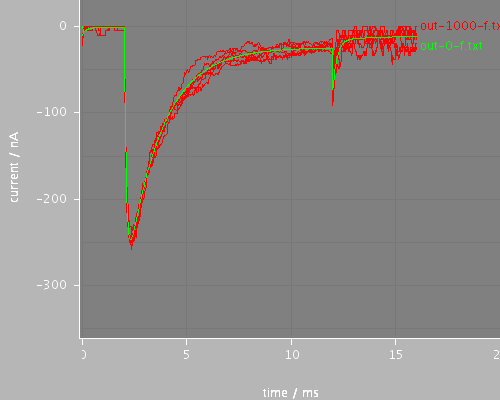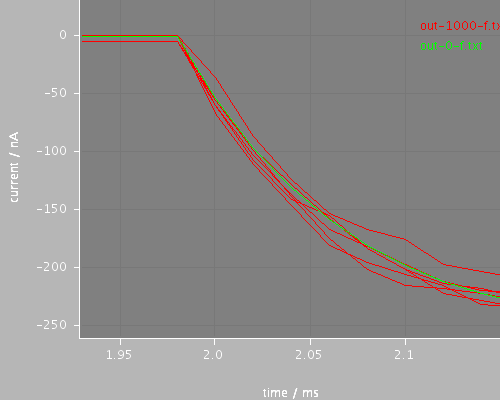## step-onset (run-onset.xml)

Voltage clamp step onset behavior for continuous and stochastic channels. The step begins at 1.985ms and the timestep is 0.02ms. This uses the midpoint evaluation method for the step, (stepStyle = midpoint in the voltage clamp) so the effect is as if the step started at 1.980ms. This fugure should be compared with the file run-onset-avg, which does the same calculation but with stepStyle = average for the clamp.

Total CPU time 0.2210 seconds; at 16:38:53 Fri 28 Sep 2007

 Compartments Stochasticchannels / cpmts Continuouschannels / cpmts time/ms timestep/ms stochThreshold CPU Time / s 1 0 / 0 201 / 1 16.0 0.02 0 0.1 1 201 / 1 0 / 0 16.0 0.02 1000 0.121### Predefined views

#### whole#### start## All files

 Model Preprocessed Outupt data Reference data etc run-onset.xml cell.xml membrane.xml environment.xml recording-short.xml na1.xml summary.xml view.xml recording-short-avg.xml out-16-fdata.txt out-0-fdata.txt out-1000-fdata.txt out-16-f.dat out-16-f.txt out-0-f.dat out-0-f.txt out-1000-f.dat out-1000-f.txt step-onset.jar

## Model

Archive file of the complete model: step-onset.jar

### run-onset.xml

```<PSICSRun timeStep="0.02ms" runTime="16ms" startPotential="-65mV"
morphology="cell"
environment="environment"
properties="membrane"
access="recording-short"
stochThreshold="0"
repeats="20">
<StructureDiscretization baseElementSize="3um"/>

<RunSet vary="stochThreshold" values="[0, 1000]"
filepattern="out-\$.txt">
</RunSet>

Voltage clamp step onset behavior for continuous and stochastic channels. The step begins
at 1.985ms and the timestep is 0.02ms. This uses the midpoint evaluation method for the step,
(stepStyle="midpoint" in the voltage clamp)
so the effect is as if the step started at 1.980ms. This fugure should be compared with
the file run-onset-avg, which does the same calculation but with stepStyle="average" for the clamp.

<ViewConfig>
<LineGraph width="500" height="400">
<XAxis min="0" max="250" label="time / ms"/>
<YAxis min="-80" max="60" label="current / nA"/>

<LineSet file="out-1000-f.txt" color="red" maxshow="6"/>
<LineSet file="out-0-f.txt" color="green" maxshow="2"/>

<View id="whole" xmin="0." xmax="20." ymin="-360." ymax="30."/>
<View id="start" xmin="1.93" xmax="2.15" ymin="-260." ymax="30."/>
</LineGraph>

</ViewConfig>

</PSICSRun>
```

### cell.xml

```<CellMorphology id="cell">

<Point id="p0" x="0" y="0" z="0" r="1."/>

</CellMorphology>
```

### membrane.xml

```<CellProperties id="membrane"
cytoplasmResistivity="100ohm_cm"
membraneCapacitance="1uF_per_cm2">

<ChannelPopulation id="napop" channel="na1" density="16per_um2"/>

</CellProperties>
```

### environment.xml

```<CellEnvironment id="environment">
<Ion id="Na" name="Sodium" reversalPotential="80mV"/>
<Ion id="K" name="Potassium" reversalPotential="-80mV"/>
</CellEnvironment>
```

### recording-short.xml

```<Access id="recording-short" saveInterval="0.05ms">

<VoltageClamp at="p0" lineColor="red" hold="-80.0mV" stepValue="midpoint">
<VoltagePulse id="vstep" start="1.985ms" to="30mV"
duration="10ms"/>
</VoltageClamp>

</Access>
```

### na1.xml

```<KSChannel id="na1" gSingle="30pS" permeantIon="Na">
<ClosedState id="c1"/>
<OpenState id="o1"/>
<ClosedState id="c2"/>

<VHalfTransition from="c1" to="o1" vHalf = "-35mV" z="4.5e" gamma="0.8" tau="0.55ms" tauMin="0.1ms"/>

<VHalfTransition from="o1" to="c2" vHalf = "-70mV" z="1.1e" gamma="0.90" tau="12.0ms" tauMin="1.5ms"/>

</KSChannel>
```

### recording-short-avg.xml

```<Access id="recording-short" saveInterval="0.05ms">

<VoltageClamp at="p0" lineColor="red" hold="-80.0mV" stepValue="average">
<VoltagePulse id="vstep" start="1.985ms" to="30mV"
duration="10ms"/>
</VoltageClamp>

</Access>
```